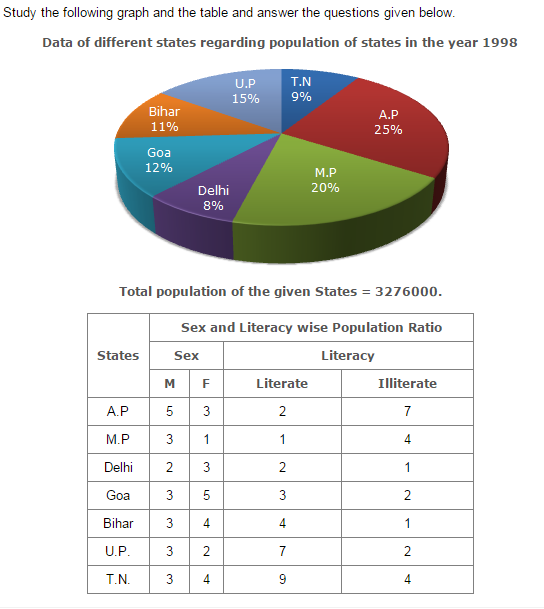Direction1.

What will be the percentage of total number of males in U.P., M.P. and Goa together to the total population of all the given states?

 A. 25% B. 27.5% C. 28.5% D. 31.5%
Explanation: Number of males in U.P = [3/5 of(15% of N)] = 3/5 X 5/100 X = 9X N/100 where N = 3276000. Number of males in M.P = [3/4 of(20% of N)] = 3/4 X 20/100 X = 15X N/100 Number of males in Goa = [3/8 of(12% of N)] = 3/8 X 12/100 X = 4.5X N/100 Therefore Total number of males in these three states = (9 + 15 + 4.5) xN/100 =(28.5 X N/100) Therefore Required Percentage = [((28.5 X 8/100)/N) X100]% =28.5%

2.

What was the total number of illiterate people in A.P. and M.P. in 1998?

 A. 876040 B. 932170 C. 981550 D. 1161160
Explanation: No. of illiterate people in A.P. = [7/9 of(25% of 3276000)]=637000 No. of illiterate people in M.P. = [4/5 of(20% of 3276000)]=524160 Therefore Total number = (637000 + 524160) = 1161160.

3.

What is the ratio of the number of females in T.N. to the number of females in Delhi?

 A. 7 : 5 B. 9 : 7 C. 13 : 11 D. 15 : 14
Explanation: Required ratio =[(4/7 of 9% of 3276000)/(3/5 of (8% of 3276000)] = [(4/7 X 9)/(3/5 X 8)] = (4/7 X 9 X 5/3 X 1/8) = 15/14

4.

What was the number of males in U.P. in the year 1998?

 A. 254650 B. 294840 C. 321470 D. 341200